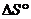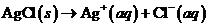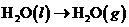Chapter 10, Problem 20ALQ### Introductory Chemistry: A Foundati...

9th Edition
Steven S. Zumdahl + 1 other
ISBN: 9781337399425

#### Solutions

Chapter
Section### Introductory Chemistry: A Foundati...

9th Edition
Steven S. Zumdahl + 1 other
ISBN: 9781337399425
Textbook Problem
1 views

# Predict the sign offor each of the following changes.l type='a'>i>i>i>Interpretation Introduction

(a)

Interpretation:

Δ S0 for the given reactions should be determined.

Concept Introduction:

Energy is the ability to do work or produce heat. The law of conservation of energy says that the energy cannot be created or destroyed, but it can be converted from one type to another. It is also called the first law of thermodynamics, according to which the energy of the universe is constant.

Entropy is a measure of uncertainty or randomness. Entropy increases as we go from solid to liquid to gas. When gas moles increases, the entropy will increase.

Explanation

The process of compressing the gas into one vessel requires work;consequently, it results in decrease in entropy...

Interpretation Introduction

(b)

Interpretation:

Δ S0 for the given reactions should be determined.

Concept Introduction:

Energy is the ability to do work or produce heat. The law of conservation of energy says that the energy cannot be created or destroyed, but it can be converted from one type to another. It is also called the first law of thermodynamics, according to which the energy of the universe is constant.

Entropy is a measure of uncertainty or randomness. Entropy increases as we go from solid to liquid to gas. When gas moles increases, the entropy will increase.

Interpretation Introduction

(c)

Interpretation:

Δ S0 for the given reactions should be determined.

Concept Introduction:

Energy is the ability to do work or produce heat. The law of conservation of energy says that the energy cannot be created or destroyed, but it can be converted from one type to another. It is also called the first law of thermodynamics, according to which the energy of the universe is constant.

Entropy is a measure of uncertainty or randomness. It increases as we go from solid to liquid to gas. When gas moles increases, the entropy will increase.

Interpretation Introduction

(d)

Interpretation:

Δ S0 for the given reactions should be determined.

Concept Introduction:

Energy is the ability to do work or produce heat. The law of conservation of energy says that the energy cannot be created or destroyed, but it can be converted from one type to another. It is also called the first law of thermodynamics, according to which the energy of the universe is constant.

Entropy is a measure of uncertainty or randomness. It increases as we go from solid to liquid to gas.When gas moles increases, the entropy will increase.

### Still sussing out bartleby?

Check out a sample textbook solution.

See a sample solution

#### The Solution to Your Study Problems

Bartleby provides explanations to thousands of textbook problems written by our experts, many with advanced degrees!

Get Started